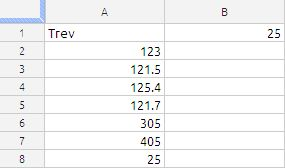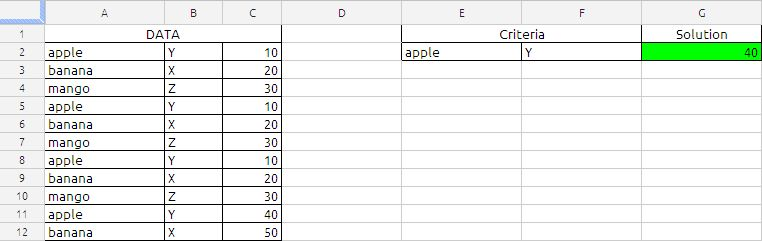## HOW TO RETRIEVE THE LAST VALUE IN A COLUMN

Suppose the data is laid as shown in the below imageThe formula in cell B1 is

=INDEX(A:A,COUNTA(A:A))

this will retrieve the value in the last cell of column A.

but the above formula will fail if the there are blank in the column A this can modified as follows:the formula in cell B1 is

=INDEX(A:A,MAX(ROW(A:A)*(A:A<>””)))

so if there are blanks in between data the above formula is written to ignore those black.

## Maximum Based on Multiple CriteriaThe Formula in cell G2 is
=ArrayFormula(MAX(IF(A2:A12=E2,IF(B2:B12=F2,C2:C12))))

the above formula will return the maximum value in cell C2:C12 corresponding to criteria specified in the cell E2 and F2.

the formula will narrows down using the conditions in the IF function first A2:A12 and then B2:B12. If the both conditions are met the resultant will be value in cell C2:C12

Which will be MAX {10,10,10,40}

based on the sample data the result will be 40.